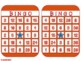# Grade 3 - Memorial Day Multiplication and Division BingoSubject
Resource Type
File Type

PDF

(21 MB|18 pages)
Product Rating
Standards
• Product Description
• StandardsNEW

Use this fun themed Bingo Game to help students practice multiplying within 100 and understanding the properties of multiplication and the relationship between multiplication and division.

Directions to the Teacher: Print and cut out Bingo cards and chips for students. Students or the teacher will use two dice and multiply the numbers to find the product. Then place a Bingo chip on the product. The first person to get 5 in a row wins.

This game can be played in partners or up to 24 players.

Fluently multiply and divide within 100, using strategies such as the relationship between multiplication and division (e.g., knowing that 8 × 5 = 40, one knows 40 ÷ 5 = 8) or properties of operations. By the end of Grade 3, know from memory all products of two one-digit numbers.
Understand division as an unknown-factor problem. For example, find 32 ÷ 8 by finding the number that makes 32 when multiplied by 8.
Apply properties of operations as strategies to multiply and divide. Examples: If 6 × 4 = 24 is known, then 4 × 6 = 24 is also known. (Commutative property of multiplication.) 3 × 5 × 2 can be found by 3 × 5 = 15, then 15 × 2 = 30, or by 5 × 2 = 10, then 3 × 10 = 30. (Associative property of multiplication.) Knowing that 8 × 5 = 40 and 8 × 2 = 16, one can find 8 × 7 as 8 × (5 + 2) = (8 × 5) + (8 × 2) = 40 + 16 = 56. (Distributive property.)
Total Pages
18 pages
N/A
Teaching Duration
N/A
Report this Resource to TpT
Reported resources will be reviewed by our team. Report this resource to let us know if this resource violates TpT’s content guidelines.